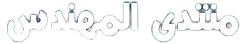# وينكم يا مهندسين بسرعه

يسعد صبااااااكم
لو سمحتووووووون ابي مسااااعده منكم بليز لاتردون
ابي حل ل هالسوال ضروري لو تقدرون لو اليوم يا مهندسين
انا بعدي طالبه

[RIGHT]The gas in the scrubber rises through a series of trays (metal plates perforated with

[RIGHT]Many small holes) and the solvent flows over the trays and through downcomers to the trays below . gas bubbles emerge from the holes in each tray and rise through the covering liquid and so2

[RIGHT]Diffuses out of the bubbles and into solution

[RIGHT]The volumetric flow rate of the feed gas is determined with an orifice

[RIGHT]Meter with adifferential mercury manometer being used to measure the pressure drop across the orifice

[RIGHT]Calibration date for this meter are tabulated here

[RIGHT]v. COLOR=#000000[/color]

[RIGHT]H(mm)

[RIGHT]142

[RIGHT]100

[RIGHT]204

[RIGHT]200

[RIGHT]247

[RIGHT]300

[RIGHT]290

[RIGHT]400

[/right]

[RIGHT]

[RIGHT]The molar density of the feed gas may be determined from the formula

[RIGHT]P(mol/liter) = 12.2p(atm)/T(k)

[RIGHT]Where p and T are the absolute pressure and temperature of the gas

[RIGHT]An electrochemical detector is used to measure the SO2 concentration in the inlet and oulet gas stream :

[RIGHT]SO2 in the sampled gas is absorbed in solution across which a fixed voltage is applied and the mole fraction of so2 in the gas is determined from the resulting current . the calibration curve for the analyzer is a straight line on a semilog plot of y(mol so2 /mol total) versus R (analyzer reading) which passes through the following points :

[RIGHT]R (rectangular scale)

[RIGHT]Y(log scale)

[RIGHT]20

[RIGHT]0.00166

[RIGHT]90

[RIGHT]0.1107

[/right]

[RIGHT]

[RIGHT]The following data are taken:

[RIGHT]T = 750 F

[RIGHT]P = 150 psig (feed gas)

[RIGHT]h (orifice meter)= 210 mm

[RIGHT]R (SO2 analyzer ) = 82.4

[RIGHT]R (so2 analyzer) =11.6 (outlet gas)

[RIGHT]Draw and completely label a process flowchart. Include in the labeling the molar flow- 1

[RIGHT]Rates and so2 mole fractions of the gas streams and the mass flow rates and so2 mass fractions of the

[RIGHT]Liquid streams . show that the scrubber has zero degrees freedom.

[RIGHT]

[RIGHT]Determine (i) the orifice meter calibration formula by plotting v. versus h on logarithmic axes and - 2

[RIGHT] (ii) the so2 analyzer calibration formula .

[RIGHT]Calculate (i) the mass fractions of so2 in the liquid effluent stream and -3

[RIGHT]COLOR=#000000 the rate at which so2 is removed from the feed gas (kg so2 /min)[/color]

[RIGHT]-The scrubber column trays commonly have diameters on the order of 1-5 meters and 4

[RIGHT]Perforation holes on the order of 4-12 mm in diameter leading to the formation of many tiny bubbles in the liquid on each tray. Speculate on the advantages of making the bubbles as small as possible.

بليز